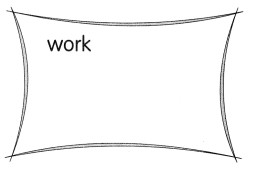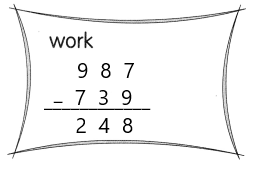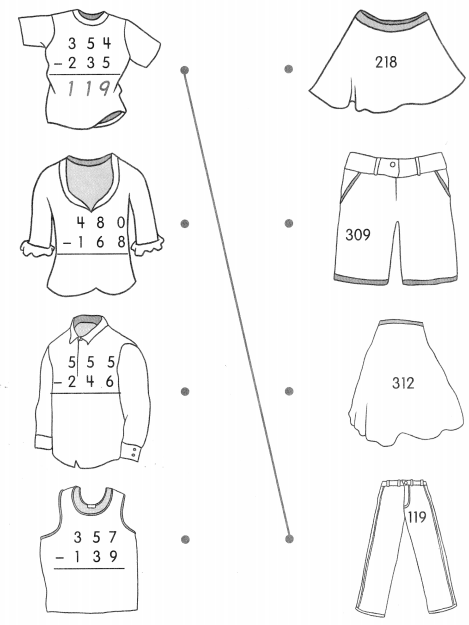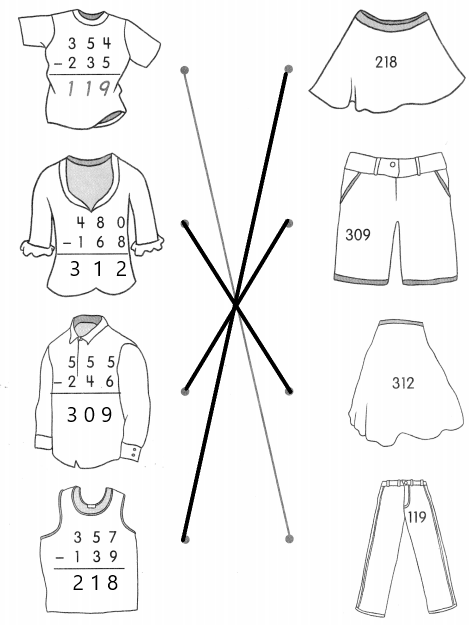# Math in Focus Grade 2 Chapter 3 Practice 3 Answer Key Subtraction with Regrouping in Tens and Ones

Go through the Math in Focus Grade 2 Workbook Answer Key Chapter 3 Practice 3 Subtraction with Regrouping in Tens and Ones to finish your assignments.

## Math in Focus Grade 2 Chapter 3 Practice 3 Answer Key Subtraction with Regrouping in Tens and Ones

Regroup the tens and ones. Then subtract.

Question 1.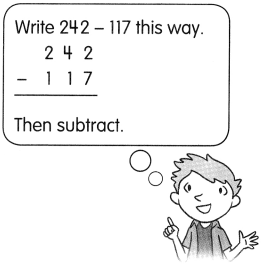242 – 117 = ?
242 – 117
= 2 hundreds 4 tens 2 ones – 1 hundred 1 ten 7 ones
= 2 hundreds 3 tens _________ ones – 1 hundred 1 ten 7 ones
= ________ hundred ________ tens ________ ones
= _____
242 – 117 = ____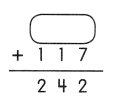242 – 117 = 125,
117+125 = 242.

Explanation:
Given that 242 – 117 which is 125. So to check the answer we will perform addition, which is
117+125 = 242.
= 2 hundreds 4 tens 2 ones – 1 hundred 1 ten 7 ones
= 2 hundreds 3 tens 12 ones – 1 hundred 1 ten 7 ones
= 1 hundred 2 tens 5 ones
= 125.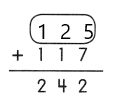Question 2.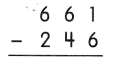661-246 = 415,
415+246 = 661.

Explanation:
Given that 661-246 which is 415. So to check the answer we will perform addition, which is
415+246 = 661.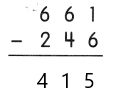Question 3.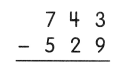743-529 = 214,
214+529 = 743.

Explanation:
Given that 743-529 which is 214. So to check the answer we will perform addition, which is
214+529 = 743.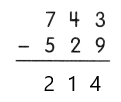Question 4.
861 – 312 = ___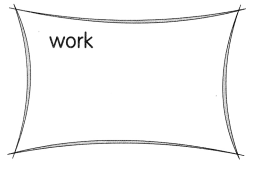861-312 = 540,
312+540 = 861.

Explanation:
Given that 861-312 which is 540. So to check the answer we will perform addition, which is
312+540 = 861.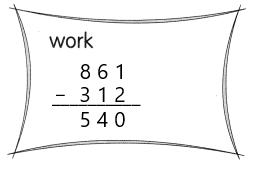Question 5.
987 – 739 = ___